## RS Aggarwal Class 7 Solutions Chapter 4 Rational Numbers Ex 4B

These Solutions are part of RS Aggarwal Solutions Class 7. Here we have given RS Aggarwal Solutions Class 7 Chapter 4 Rational Numbers Ex 4B.

Other Exercises

Question 1.
Solution:
(i) Draw a number line and locate a point O on it. Let it represent 0 Now $$\frac { 1 }{ 3 }$$ has been presented on the number line given below.(ii) Draw a number line and locate a point O on it. Let it represent 0. The number $$\frac { 2 }{ 7 }$$ has been represented on the number line given below:(iii) Draw a number line and locate a point O on it. Let it represent 0. The number $$\frac { 7 }{ 3 }$$ has been represented on the number line given below: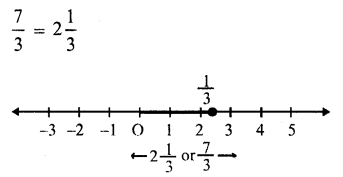(iv) Draw a number line and locate a point O on it. Let it represent 0. The number $$\frac { 7 }{ 3 }$$ has been represented on it as given below: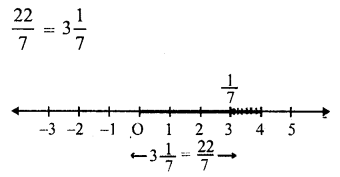(v) Draw a number line and locate a point O on it. Let it represented 0. The number $$\frac { 37 }{ 8 }$$ has been represented on it as given below:
$$\frac { 37 }{ 8 }$$ = 4$$\frac { 5 }{ 8 }$$(vi) Draw a number line and locate a point O on it. Let it represent 0. The number $$\frac { -1 }{ 3 }$$ has been represented on it as given below: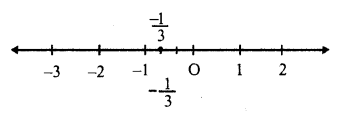(vii) Draw a number line and locate a point O on it. Let it represent 0. The number $$\frac { -3 }{ 4 }$$ has been represented on it is as given below: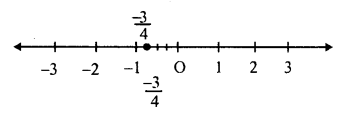(viii) Draw a number line and locate a point on it. Let it represent 0. The number $$\frac { -12 }{ 7 }$$ has been represented on it as given below: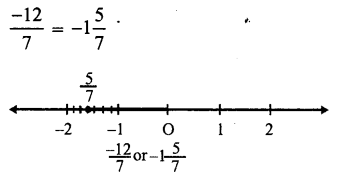(ix) Draw a number line and locate a point O on it. Let it represent 0. The number $$\frac { 36 }{ -5 }$$ has been represented on it as given below: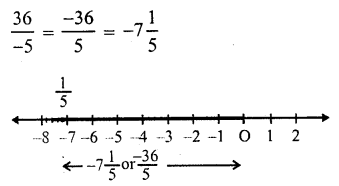(x) Draw a number line and locate is point O on it. Let is represent 0. The number $$\frac { -43 }{ 9 }$$ has been represented on it as given below: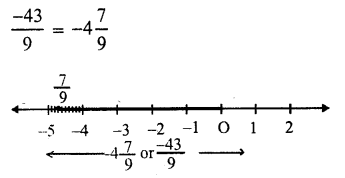Question 2.
Solution:
(i) $$\frac { 5 }{ 6 }$$ or 0, $$\frac { 5 }{ 6 }$$ is greater as any positive number is always greater than 0.Question 3.
Solution: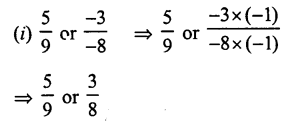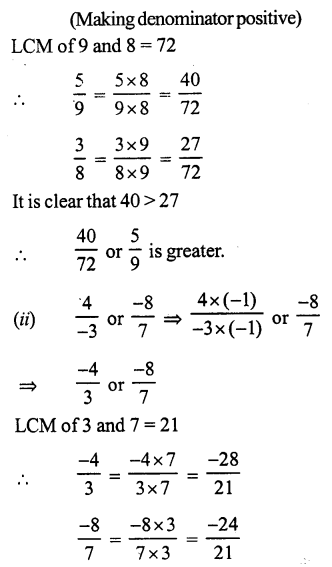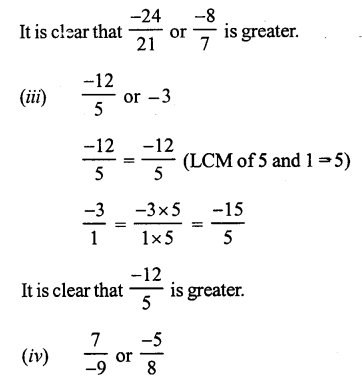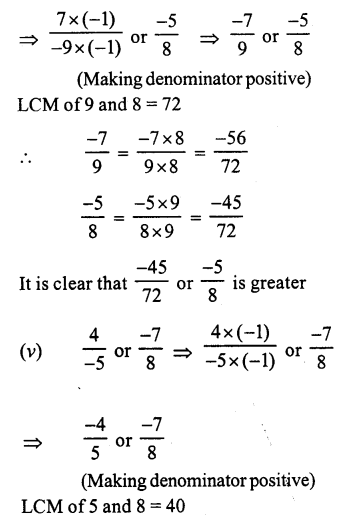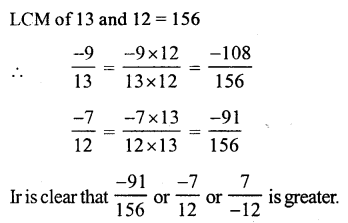Question 4.
Solution: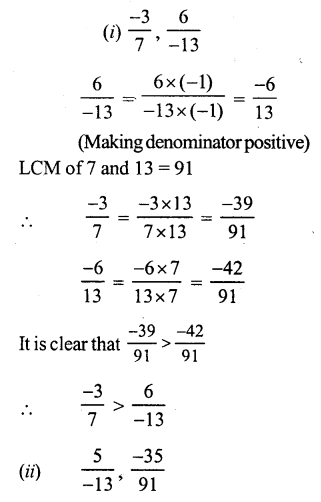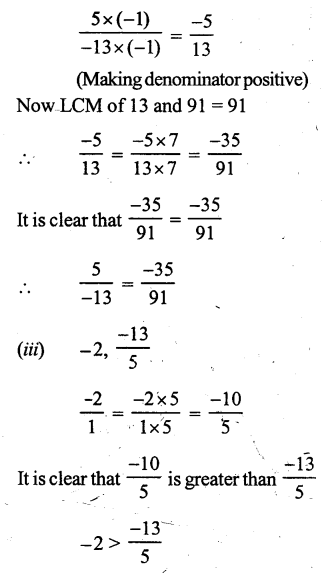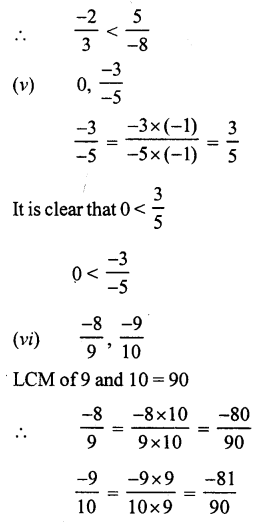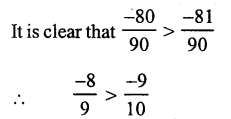Question 5.
Solution: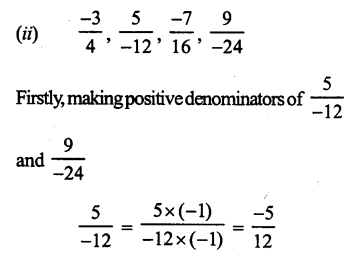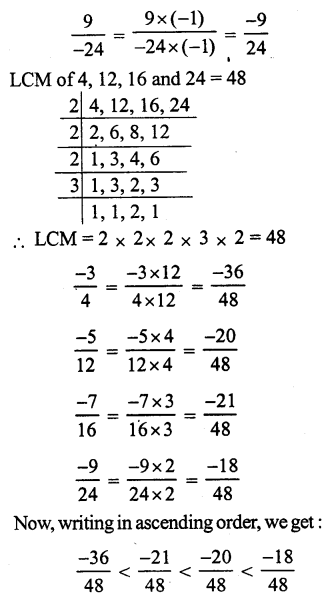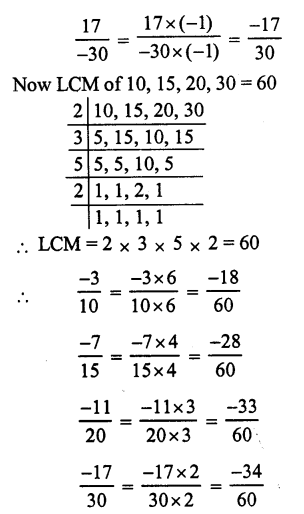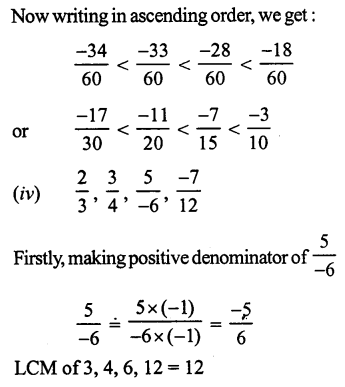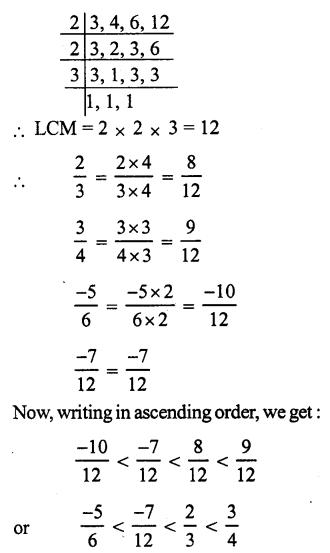Question 6.
Solution: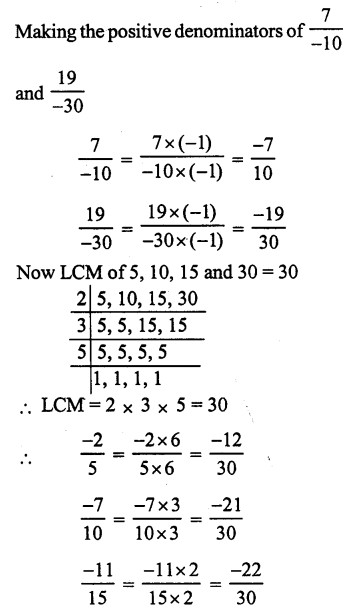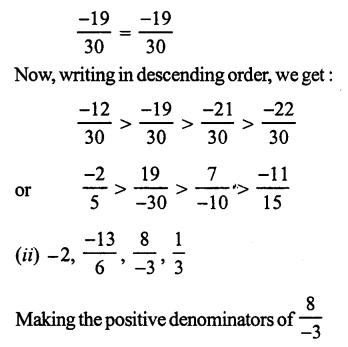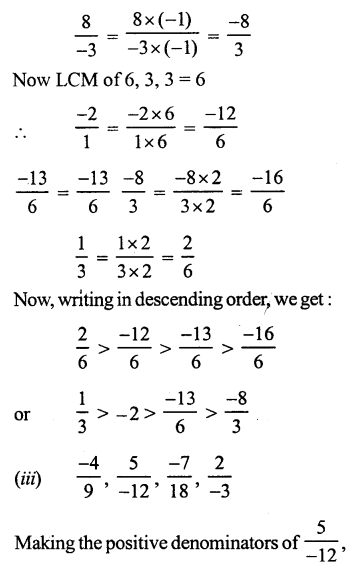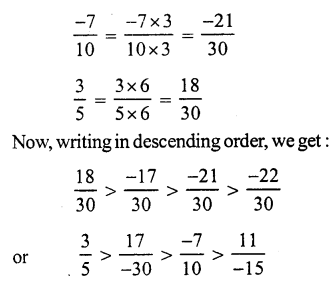Question 7.
Solution:
(i) True: All negative numbers lie on the left of 0.
(ii) False: All negative numbers lie on the left of 0.
(iii) True: All positive numbers lie on the right of 0 and all negative numbers on the left of 0.
(iv) False: $$\frac { -18 }{ -13 }$$ = $$\frac { 18 }{ 13 }$$ which is positive and positive number lie on the left of 0.
(v) True: $$\frac { -5 }{ -8 }$$ = $$\frac { 5 }{ 8 }$$ which is positive and all positive number lie on the right of negative numbers.
(i), (iii) and (iv) are true.

Question 8.
Solution:
5 rational numbers between -3 and -2.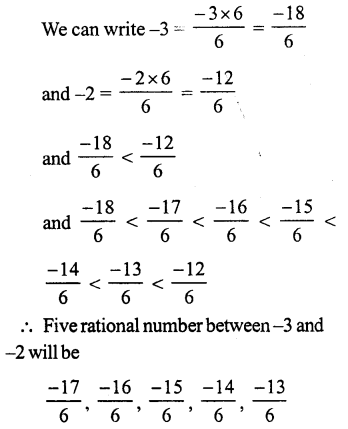Question 9.
Solution: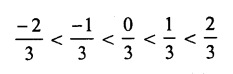Question 10.
Solution:
L.C.M. of 5 and 2 = 10Hope given RS Aggarwal Solutions Class 7 Chapter 4 Rational Numbers Ex 4B are helpful to complete your math homework.

If you have any doubts, please comment below. Learn Insta try to provide online math tutoring for you.